• +91 9971497814
• info@interviewmaterial.com

# RD Chapter 12- Heron-s Formula Ex-MCQS Interview Questions Answers

### Related Subjects

Question 1 :
In ∆ABC ≅ ∆LKM, then side of ∆LKM equal to side AC of ∆ABC is
(a) LX
(b) KM
(c) LM
(d) None of these

Answer 1 : Side AC of ∆ABC = LM of ∆LKM (c)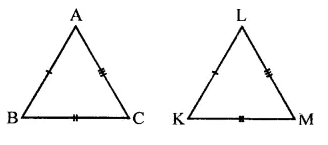Question 2 :
In ∆ABC ≅ ∆ACB, then ∆ABC is isosceles with
(a) AB=AC
(b) AB = BC
(c) AC = BC
(d) None of these

∵ ∆ABC ≅ ∆ACB
∴ AB = AC (a)

Question 3 :
In ∆ABC ≅ ∆PQR, then ∆ABC is congruent to ∆RPQ, then which of the following is not true:
(a) BC = PQ
(b) AC = PR
(c) AB = PQ
(d) QR = BC

∵ ∆ABC = ∆PQR
∴ AB = PQ, BC = QR and AC = PR
∴ BC = PQ is not true (a)

Question 4 :
In triangles ABC and PQR three equality relations between some parts are as follows: AB = QP, ∠B = ∠P and BC = PR State which of the congruence conditions applies:
(a) SAS
(b) ASA
(c) SSS
(d) RHS

In two triangles ∆ABC and ∆PQR,
AB = QP, ∠B = ∠P and BC = PR
The condition apply : SAS (a)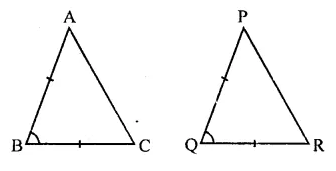Question 5 :
In triangles ABC and PQR, if ∠A = ∠R, ∠B = ∠P and AB = RP, then which one of the following congruence conditions applies:
(a) SAS
(b) ASA
(c) SSS
(d) RHS

In ∆ABC and ∆PQR,
∠A = ∠R
∠B = ∠P
AB = RP
∴ ∆ABC ≅ ∆PQR (ASA axiom) (b)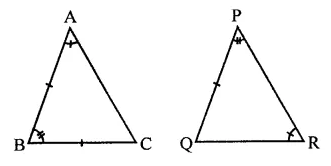Question 6 :
If ∆PQR ≅ ∆EFD, then ED =
(a) PQ
(b) QR
(c) PR
(d) None of these

∵ ∆PQR = ∆EFD
∴ ED = PR (c)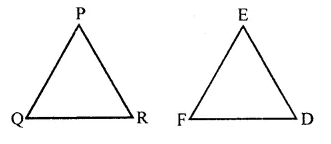Question 7 :
If ∆PQR ≅ ∆EFD, then ∠E =
(a) ∠P
(b) ∠Q
(c) ∠R
(d) None of these

∵ ∆PQR ≅ ∆EFD
∴ ∠E = ∠P (a)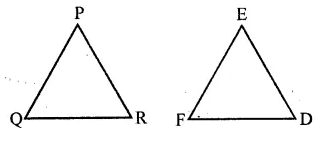Question 8 :
In a ∆ABC, if AB = AC and BC is produced to D such that ∠ACD = 100°, then ∠A =
(a) 20°
(b) 40°
(c) 60°
(d) 80°

In ∆ABC, AB = AC
∴ ∠B = ∠C
But Ext. ∠ACD = ∠A + ∠B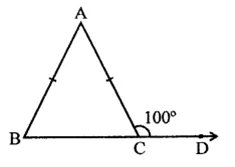∠ACB + ∠ACD = 180° (Linear pair)
∴ ∠ACB + 100° = 180°
⇒ ∠ACB = 180°-100° = 80°
∴ ∠B = ∠ACD = 80°
But ∠A + ∠B 4- ∠C = 180°
∴ ∠A + 80° + 80° = 180°
⇒∠A+ 160°= 180°
∴ ∠A= 180°- 160° = 20° (a)

Question 9 :
In an isosceles triangle, if the vertex angle is twice the sum of the base angles, then the measure of vertex angle of the triangle is
(a) 100°
(b) 120°
(c) 110°
(d) 130°

In ∆ABC,
∠A = 2(∠B + ∠C)
= 2∠B + 2∠C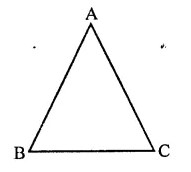∠A + 2∠A = 2∠A + 2∠B + 2∠C
⇒ 3∠A = 2(∠A + ∠B + ∠C)
⇒ 3∠A = 2 x 180° (∵∠A + ∠B + ∠C = 180° )
⇒ 3∠A = 360°
⇒∠A =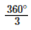= 120°
∴ ∠A = 120° (b)

Question 10 :
Which of the following is not a criterion for congruence of triangles?
(a) SAS
(b) SSA
(c) ASA
(d) SSS

Answer 10 : SSA is not the criterion of congruence of triangles. (b)

Todays Deals### RD Chapter 12- Heron-s Formula Ex-MCQS Contributorskrishan

Name:
Email:

# Latest News# 9000 interview questions in different categories